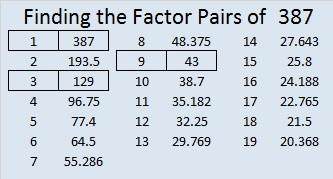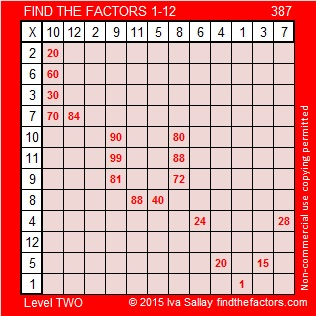# 387 and Level 2

Is 387 prime or composite? Well, 8 + 7 = 15, a multiple of 3, so 387 is a composite number divisible by 3.

Here’s sending a little luv your way!

Print the puzzles or type the factors on this excel file:12 Factors 2015-02-09

• 387 is a composite number.
• Prime factorization: 387 = 3 x 3 x 43, which can be written 387 = (3^2) x 43
• The exponents in the prime factorization are 2 and 1. Adding one to each and multiplying we get (2 + 1)(1 + 1) = 3 x 2  = 6. Therefore 387 has exactly 6 factors.
• Factors of 387: 1, 3, 9, 43, 129, 387
• Factor pairs: 387 = 1 x 387, 3 x 129, or 9 x 43
• Taking the factor pair with the largest square number factor, we get √387 = (√9)(√43) = 3√43 ≈ 19.672This site uses Akismet to reduce spam. Learn how your comment data is processed.